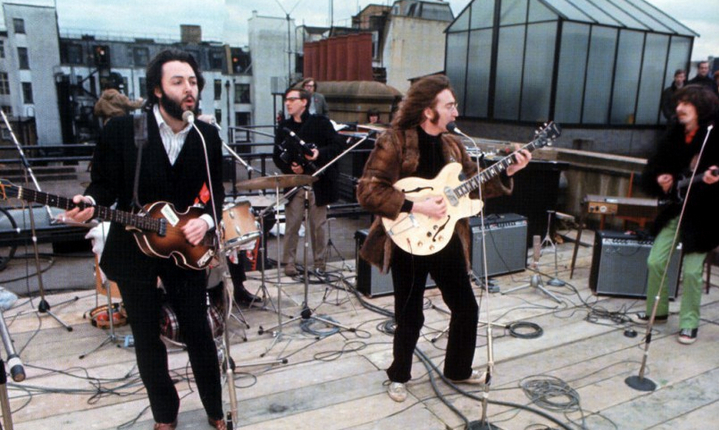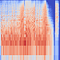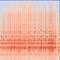# Using Convolutional Neural Networks And Scikit-Optimize To Predict Who Had The Largest Influence Within The BeatlesThe Beatles’ London rooftop concert, 1969

# Data CollectionMel Spectrogram image of the song “A Day In The Life” off Sgt. Pepper’s Lonely Hearts Club

# Data ProcessingShort-Time Fourier Transform of “Working Class Hero” by John Lennon

# Interpreting the Results

`from model_staging import fetch_images_dataframeimport pandas as pdimport numpy as npimport kerastrain_df = pd.read_csv("train_df.csv")test_df = pd.read_csv("test_df.csv")train_path = "C://Users//Alec//MyPython//Beatles/train_melspec"test_path = "C://Users//Alec//MyPython//Beatles/test_melspec"# load the Beatles data to predict ontest_gen = fetch_images_dataframe(test_df, x_col="song",                                   y_col="artist",                                   directory=test_path,                                  batch_size=16,                                   target_size=(256, 256),                                   class_mode="categorical",                                   shuffle=False, seed=1,                                   save_format="png")# load the modelbest_model_adam = keras.models.load_model("models/melspec/skopt_best_adamV3.h5")# predict the probabilities for each songprobabilities = best_model_adam.predict_generator(test_gen)# get the prediction based on largest probabilitypreds = np.argmax(probabilities, axis=1)# load in the training data to get the artist indiciestrain_gen, valid_gen = fetch_images_dataframe(train_df,                                               x_col="song",                                               y_col="artist",                                               directory=train_path,                                              batch_size=16,                                               target_size=(256, 256),                                               class_mode="categorical",                                               shuffle=True, seed=1,                                               validation_split=0.2,                                               save_format="png")class_map = train_gen.class_indices# create a dataframe of songs and predictionspred_df = pd.DataFrame(data={"songs": test_gen.filenames,                                   "predictions": preds})# now convert the prediction column to the artist namemapping = {v:k for k,v in class_map.items()}pred_df["predictions"] = pred_df["predictions"].map(mapping)# merge the pred_df with the test_df in order to bring the album# name in for each songpred_df = pred_df.merge(test_df[["album", "song"]], left_on="songs",right_on="song")pred_df.drop("song", axis=1, inplace=True)# join the prediction probabilities with the prediction dataframepred_df = pred_df.join(pd.DataFrame(probabilities))pred_df.rename(mapping, axis=1, inplace=True)pred_df[['Lennon', 'harrison', 'mccartney', 'starr']] = pred_df[['Lennon', 'harrison', 'mccartney', 'starr']].round(4)`

# Conclusion and Lessons Learned

Applied Data Science Master's Student. Data Science and finance enthusiast,

## More from Alec Francis

Applied Data Science Master's Student. Data Science and finance enthusiast,